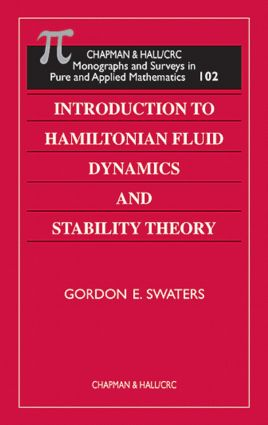# Introduction to Hamiltonian Fluid Dynamics and Stability Theory

## 1st Edition

Chapman and Hall/CRC

288 pages

##### Purchasing Options:\$ = USD
Hardback: 9781584880233
pub: 1999-09-29
SAVE ~\$37.00
\$185.00
\$148.00
x
eBook (VitalSource) : 9780203750087
pub: 2019-01-22
from \$103.77

FREE Standard Shipping!

### Description

Hamiltonian fluid dynamics and stability theory work hand-in-hand in a variety of engineering, physics, and physical science fields. Until now, however, no single reference addressed and provided background in both of these closely linked subjects. Introduction to Hamiltonian Fluid Dynamics and Stability Theory does just that-offers a comprehensive introduction to Hamiltonian fluid dynamics and describes aspects of hydrodynamic stability theory within the context of the Hamiltonian formalism.

The author uses the example of the nonlinear pendulum-giving a thorough linear and nonlinear stability analysis of its equilibrium solutions-to introduce many of the ideas associated with the mathematical argument required in infinite dimensional Hamiltonian theory needed for fluid mechanics. He examines Andrews' Theorem, derives and develops the Charney-Hasegawa-Mima (CMH) equation, presents an account of the Hamiltonian structure of the Korteweg-de Vries (KdV) equation, and discusses the stability theory associated with the KdV soliton.

The book's tutorial approach and plentiful exercises combine with its thorough presentations of both subjects to make Introduction to Hamiltonian Fluid Dynamics and Stability Theory an ideal reference, self-study text, and upper level course book.

### Reviews

"…a refreshingly non-technical style…this is a well-written introduction to Hamiltonian fluid dynamics and basic stability results."

--S. Reich, Edinburgh Mathematical Society, Vol. 44

Introduction

The Nonlinear Pendulum

Model Formulation

Canonical Hamiltonian Formulation

Least Action Principle

Symplectic Hamiltonian Formulation

Mathematical Properties of the J Matrix

Poisson Bracket Formulation

Steady Solutions of a Canonical Hamiltonian System

Linear Stability of a Steady Solution

Nonlinear Stability of a Steady Solution

The Two Dimensional Euler Equations

Vorticity Equation Formulation

Hamiltonian Structure for Partial Differential Equations

Hamiltonian Structure of the Euler Equations

Reduction of the Canonical Poisson Bracket

Casimir Functionals and Noether's Theorem

Exercises

Stability of Steady Euler Flows

Steady Solutions of the Vorticity Equation

Linear Stability Problem

Normal Mode Equations for Parallel Shear Flows

Linear Stability Theorems

Nonlinear Stability Theorems

Andrews' Theorem

Flows with Special Symmetries

Exercises

The Charney-Hasegawa-Mima Equation

A Derivation of the CHM Equation

Hamiltonian Structure

Stability of Steady Solutions

Exercises

The KdV Equation

A Derivation of the KdV Equation

Hamiltonian Structure

Periodic and Soliton Solutions

Variational Principles

Linear Stability Nonlinear Stability

Exercises

### Subject Categories

##### BISAC Subject Codes/Headings:
MAT000000
MATHEMATICS / General
MAT003000
MATHEMATICS / Applied
MAT007000
MATHEMATICS / Differential Equations
SCI040000
SCIENCE / Mathematical Physics